# High speed Radix-4 Booth scheme in CNTFET technology for high performance parallel multipliers

Document Type : Reasearch Paper

Authors

1 Department of Electrical Engineering, Rasht Branch, Islamic Azad University, Rasht, Iran.

2 Department of Electrical and Computer Engineering, University of Mohaghegh Ardabili, Ardabil, Iran.

Abstract

A novel and robust scheme for radix-4 Booth scheme implemented in Carbon Nanotube Field-Effect Transistor (CNTFET) technology has been presented in this paper. The main advantage of the proposed scheme is its improved speed performance compared with previous designs. With the help of modifications applied to the encoder section using Pass Transistor Logic (PTL), the corresponding capacitances of middle stages have been reduced considerably. As a result, total transistor count along with power consumption has been decreased illustrating the other advantages of the designed structure. For evaluation of correct functionality, simulations using CNTFET 32nm standard process have been performed for the designed scheme which depict the latency of 195ps for critical path. Meanwhile, comparison with previous works using the Power Delay Product (PDP) criteria demonstrates the superiority of the proposed structure suggesting that our circuitry can be widely utilized for high speed parallel multiplier design.

Keywords20.1001.1.20088868.2019.10.3.6.1

Main Subjects

#### Full Text

INTRODUCTION

Having the diameter size in the scale of nanometer which can change up to a few micrometers in length, Carbon Nanotubes (CNTs) contain one-dimensional tubular structure , which are introduced first by Iijima in 1991 . In this presented model, thick graphene sheet has been used to define the CNT and based on the number of layers; different categories of CNTs can be specified . For consumer electronics, Single-Wall CNTs (SWCNTs) showed good promise to manufacture the next generation of Integrated Circuits (ICs) because of their unique structural properties . However, the first reliable implementation of CNT Field-Effect Transistor (CNTFET) circuits is achieved about 15 years after Iijima’s invention .

A brief review of state-of-the-art works in the field of CNTFETs demonstrate that wide range of electronic devices involving low power digital electronics [6, 7] are nowadays being fabricated using CNTs to benefit from the low power and high speed characteristics of the CNTFET devices. The CNTFET device can be utilized in the implementation of the high performance microprocessors, too. One of the basic building blocks of modern microprocessors is the parallel multiplier which lies in the critical path for delay of the block and directly determines the power and speed performance of such systems .

Because of their higher performance, parallel multipliers are the design choice for circuit designers , although their building blocks are more complicated than their serial counterparts . Among the different procedures utilized for implementation of a parallel multiplier, radix-4 Booth algorithm is one of the popular structures due to its unique capability for reduction of the Partial Products (PPs) at the first stage of multiplication process . Knowing that a general purpose parallel multiplier has been composed of three main stages , including Partial Product Generation (PPG) block, Partial Product Reduction Tree (PPRT), and the final adder stage, the circuitry pertaining to Booth algorithm constitutes the first stage of multiplication chain in which the PPs are determined by means of this procedure.

Although there are several works for hardware realization of Booth algorithm in the literature [12-18], all of them have been designed in Complementary Metal-Oxide-Semiconductor (CMOS) submicron technologies. Among these works, comparative analysis shows that in [13-15] the operation speed was targeted as the main factor while the power optimization was the primary emphasis in [12, 16-18], both parameters were the subject of improvement where the cost paid was a complicated error-tolerant system.

The main idea behind this article is based on a previous work by the authors  in which a novel low power scheme was presented for implementation of the second stage of a parallel multiplier consisting of 4-2 compressors. Following that work, in this paper, a robust scheme has been presented for radix-4 Booth algorithm in CNTFET technology, which depicts better specifications in comparison with the previous designs. In order to demonstrate the advantage of the proposed structure, the best reported works in literature have been redesigned and simulated here in CNTFET technology for fair comparison.

The organization of manuscript is as follows. In section 2 of the paper, the design of the proposed architecture has been discussed, while the simulation results and comparative analysis were explained in section 3. The conclusions will be given in section 4 of the paper.

EXPERIMENTALS

Along with the introduction of the term Parallel Multiplier by Arthur Robinson , the middle years of the 20th century has faced an evolution of different procedures for fast multiplication including Booth , Karatsuba , Wallace  and Dadda  algorithms. Among these methods, Booth algorithm has attracted the attention of circuit designers over recent years because of its exciting properties for effective coding of binary numbers, which could lead to the reduction of PPs in a multiplier array.

By defining A as the first number known as multiplicand and B as the second number denoted as multiplier , the binary representation of these numbers can be expressed as: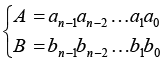(1)

where ai and bi illustrate each bit in binary basis. With the help of Booth algorithm and by decomposing B to its adjacent pair of bits, the multiplication of A and B can be written in the form of: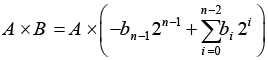(2)

in which b-1 = 0 and n represent the maximum number of bits for both numbers. Equation (2) is well known as radix-2 Booth multiplication routine, which can multiply two signed binary numbers. By modifying B as is presented in  and rewritten herein Eq. (3):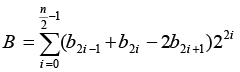(3)

and defining: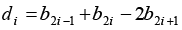(4)

as the radix-4 sign digit representation coefficient, the substitution of (3) and (4) in (2) results in: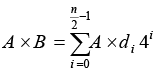(5)

Now, comparison of (2) and (5) demonstrates that employing such coding which is known as radix-4 Booth algorithm, the number of PPs (defined by n) are almost halved. Therefore, huge speed enhancement is expected if this method is utilized for the hardware implementation of a parallel multiplier. Meanwhile, the coefficient di in Eq. (5) is an indication of scale factors (-2X, -X, 0, X, 2X) for multiplication process. Table 1 summarizes the conventional truth table used for radix-4 Booth scheme implementation.

The architectures reported in [12-13] have utilized the conventional truth table for implementation of their circuits. However, none of them could reach latencies less than 4 XOR logic gates. On the other hand, the structures in [14-15] have introduced new truth tables which were the extended version of the general truth table. The result of this modification was the achievement of 2 XOR logic gate level delays from inputs to the outputs.

The main novelty of the design proposed in [14-15] was the elimination of 2X scale factor inside the encoding stage. They instead introduced one or two new intermediate parameters which led to the implementation of high speed architecture. One of the common parameters among all previously presented works is the sign bit factor which is denoted as Neg. An in-depth view of the proposed ideas depicts that simpler structures can be obtained for the radix-4 Booth encoding-decoding scheme. Considering Table 2 which was previously reported in , it is clear that the parameter  equals to: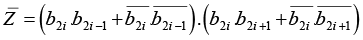(6)

Boolean logic simplification of (6), results in: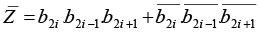(7)

which demonstrates that when all three inputs have the same state,  will have high logic value. With the help of (7) the circuit level implementation of encoder section has been illustrated in Fig. 1. In order to explain the design concept behind this structure, one can say that if ,  will be equal to  and this situation has been realized with the help of three transistors in series. Following the same procedure, if , then another branch consisting of three transistors in series can produce  output. Moreover, when the logic state of scaling factor  becomes zero, we have  and a single NMOS transistor will be enough to produce the corresponding output.

To reduce total transistor count, the XOR gates which are generating X1 and X2 output can be implemented with non-full swing four transistor gates proposed in  since they are driving the gates having only NMOS transistor. Compared to design reported in  which has employed 10 transistor XOR/XNOR gates originally reported in , all of the full swing XOR and XNOR gates in this work have been implemented by means of 6 transistor gates described in . Fig. 2 shows the redesigned XOR gates in CNTFET technology which are utilized in our scheme.

Moreover, the PMOS transistors that are fed by the inverted state of the inputs can be replaced by NMOS counterparts to save six transistors already used including those inside the inverters. By applying these techniques, the architecture of Fig. 3 will be obtained as the improved version of the proposed Booth encoder.

Compared with the circuitry of , two transistors have been reduced in the architecture of Booth encoder and the latency is reduced to less than two XOR gates which illustrates the advantages of proposed encoder section. For the decoder section, the circuit of Fig. 4 has been utilized which is derived from  employing the CNTFET technology in which the full swing XOR gates of Fig. 2 were employed.

To calculate gate-level delay from inputs to the outputs, it is clear that the critical path starts from encoder section where the parameter Z is being generated and ends in the PP where the gate of final Transmission Gate (TG) is fed by Z signal. Compared with , which has a gate level delay equal to two XOR logic gates plus one transistor, in the proposed scheme the delay has been reduced to less than two XOR gates considering the fact that the propagation delay for Z is equal to one XOR gate plus one inverter. In , although the gate level latency is claimed to be one XOR gate plus one transistor, the inverted state of the PP is obtained at the output node which needs an extra inverter to get the PP itself. Besides, in the design reported in , four parallel paths were used to produce the output while in the proposed architecture this problem is clearly improved by using two paths in parallel.

RESULTS AND DISCUSSIONS

1. Propagation Delay Evaluation

In order to have a better insight into the advantages of the proposed radix-4 scheme, the Elmore delay rule  has been employed to evaluate the propagation latency. By means of Elmore method, the time constants for different paths are examined and the largest value of the calculated time constant will determine the critical path delay. The corresponding propagation latency when the output signal reaches 50% of its final value will be 69% of the relevant time constant.

Considering the critical path for Z, the schematic of Fig. 5 can be used for calculation of delay in which Fig. 5(a) demonstrates decomposition of the path for generation of , while Fig. 5(b) shows the corresponding path for Z, X1, and X2 (because of having similar structure). Fig. 5(c) illustrates the decomposition of the decoder section in which CDiff denotes the diffusion capacitance; since it has a minimal value, therefore, has been neglected in calculations.

By defining Cinv and CTG as the corresponding capacitances for inverter and TG, respectively and by assuming that Cinv = CTG, it is clear that there are two parallel paths for signal propagation. The first path starts from the inputs, produces  and Z and faces one TG towards the output node. In the second path, the input signals propagate through one of the XOR gates (via X1 or X2) to the decoder circuit and reach the output node by passing through two TGs.

For the first path, the delay will be obtained by summing three individual time constants denoted by,  and .  represents the time constant for the generation of  and based on the architecture of Fig. 5(a) is equal to :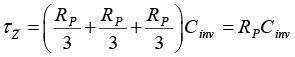(8)

where in this equation, RP defines the equivalent resistance of PMOS transistor. For  which describes the time constant in which Z signal is being created, we have: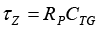(9)

Finally,  illustrates the time constant for signal propagation through a TG, which is equal to: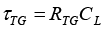(10)

which in this equation CL characterizes the load capacitance. Summation of these latencies with the assumption of Cinv = CTG and RP = RTG, results in the propagation delay of the first path which is equal to: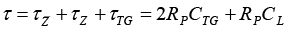(11)

By applying the same procedure to the second path, the delay will be the summation of two time constants  and , where  represents the time constant for either X1 and X2 outputs and  illustrates the time constant for decoder section . With the help of Fig. 5(b)  can be written as: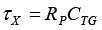(12)

By using Fig. 5(c) for the decoder section, the  parameter is calculated as :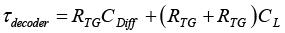(13)

and because CDiff << CL and RP = RTG, then (13) will be simplified as: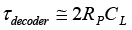(14)

By adding these latencies, the propagation delay for the second path can be obtained which is equal to: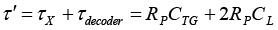(15)

Considering the fact that the load capacitance will be the input capacitance of the PPRT in a parallel multiplier, CL will has a value much greater than CTG. As a result,  , and the critical path will be determined by the second path.

1. Simulation Results

In order to measure the delay of the proposed radix-4 Booth scheme, the simulations have been carried out in HSPICE using the CNTFET32nm standard process having 0.6V power supply. Fig. 6 demonstrates the results, which indicate the correct functionality of the proposed architecture and shows a delay of about 195ps.

For a fair comparison between the proposed Booth scheme and the recently reported distinguished works in this field, the circuits reported in , ,  and  are simulated in similar conditions along with the proposed design in this work to obtain the delay and power consumption of these designs. To achieve this, the same gates including the XOR gates of Fig. 2 were used in the architectures of simulated works while a capacitive load consisting of the 4-2 compressors from  was employed to provide a more realistic environment. The results, which are shown in Fig. 7 and Fig. 8 for the delay and power comparison, respectively, illustrate that the proposed Booth structure has less delay than the previous works; however, the design reported in  has the smallest power consumption.

Table 3 summarizes the comparison results based on simulations in CNTFET 32nm process. To interpret the results realistically, the Power Delay Product (PDP) was calculated for all these designs and is also added to the table for a better comparison. The PDP specification illustrates that our work has better performance than previous designs. It must be mentioned that all simulations are performed at the operating frequency of 100MHz.

To investigate the correct behavior of the proposed circuit under different operating conditions, the temperature has been swept from -20⁰C to 120⁰C in CNTFET technology and the results have been shown in Fig. 9. Fig. 9(a) illustrates variations of the latency of critical path while the curvature of power dissipation versus temperature changes is presented in Fig. 9(b).

Finally, the supply voltage has been swept from 0.5V to 1.0V to demonstrate the changes in delay along with the variations of power supply. The result which is shown in Fig. 10 illustrates that the supply increment reduces the critical path delay.

CONCLUSIONS

In this manuscript, a novel and robust scheme for radix-4 Booth scheme has been presented which outperforms the previous works from the viewpoint of speed performance. Proposed in CNTFET technology, the main advantages of the proposed scheme are its improved speed performance and power-delay efficient feature which makes it a very potential candidate to be used inside the high performance parallel multipliers. These improvements have been achieved by modifications applied to the encoder section using PTL which led to the decrement of middle stage capacitances while the analytic calculations show conformity with design considerations.

For evaluation of correct functionality, simulations using CNTFET 32nm standard process have been performed for the designed scheme, which depicts the latency of 195ps for the critical path. In addition, the comparison with previous works using PDP specification demonstrates the superiority of proposed structure over previous designs.

CONFLICT OF INTEREST

The authors declare that there are no conflicts of interest regarding the publication of this manuscript.

#### References

           Liu C., Cheng H., (2013), Carbon nanotubes: controlled growth and application. Materials Today. 16: 19-28.
           Iijima S., (1991), Helical microtubules of graphitic carbon. Nature. 354: 56–58.
           Dresselhaus M. S., Dresselhaus G., Saito R., (1995), Physics of carbon nanotubes. Carbon. 33: 883-891.
          Avouris P., Afzali A., Appenzeller J., Chen J., Freitag M., Klinke C., Lin Y.-M., Tsang J. C., (2004), Carbon nanotube electronics and optoelectronics. Technical Digest - IEEE Int. Electron Dev.Meeting (IEDM). 12: 525-529.
           Franklin A. D., (2013), Electronics: The road to carbon nanotube transistors. Nature. 498: 443-444.
          Sedaghat M., Salimi M., (2015), Evaluation and comparison of CMOS logic circuits with CNTFET. UCT J. Res. Sci. Eng. Technol. 3: 1-9.
           Moaiyeri M. H., Rahi A., Sharifi F., Navi K., (2017), Design and evaluation of energy-efficient carbon nanotube FET-based quaternary minimum and maximum circuits. J. Appl. Res. Technol. 15: 233–241.
           Parhami B., (2000), Computer Arithmetic. Oxford Press.
          Parhi K., (1999), VLSI Digital Signal Processing Systems: Design and Implementation, Wiley-Interscience. 1st edition.
        Ohkubo N., Suzuki M., Shinbo T., Yamanaka T., Shimizu A., Sasaki K., Nakagome Y., (1995), A 4.4 ns CMOS 54 54-b multiplier using pass-transistor multiplexer. IEEE J. Solid-State Circuits. 30: 251-257.
        Aliparast P., Koozehkanani Z. D., Nazari F., (2013), An ultra high speed digital 4-2 compressor in 65-nm CMOS. Int. J. Computer Theory Eng.5: 593-597.
        Kuang S.-R., Wang J.-P., Guo C.-Y., (2009), Modified booth multipliers with a regular partial product array. IEEE Transact. Circuits Systems—II: Express Briefs. 56: 404-408.
        Rajput R., Swamy M. N., (2012), High speed modified booth encoder multiplier for signed and unsigned numbers. 14th Int. Conf. Modelling and Simul. 649-654.
        Fathi A., Azizian S., Fathi R., Tamar H. G., (2012), Low latency, glitch-free booth encoder-decoder for high speed multipliers. IEICE Electron. Express. 9: 1335-1341.
        Fathi A., Azizian S., Hadidi Kh., Khoei A., (2012), Ultra high speed modified booth encoding architecture for high speed parallel accumulations. IEICE Transact. Electron. 95: 706-709.
        Kumre L., Somkuwar A., Agnihotri G., (2013), Implementation of radix 4 booth multiplier using MGDI technique. AICERA/ICMiCR Conference.
       Nagamani A. N., Nikhil R., Nagaraj M., Agrawal V. K., (2016), Reversible radix-4 booth multiplier for DSP applications. Int. Conf. Signal Process. Communicat. (SPCOM).
        Liu W., Qian L., Wang Ch., Jiang H., Han J., Lombardi F., (2017), Design of approximate radix-4 booth multipliers for error-tolerant computing. IEEE Transact. Comput. 66:1435-1441.
        Rahnamaei A., Zare Fatin Gh., Eskandarian A., (2019), Design of a low power high speed 4-2 compressor using CNTFET 32nm technology for parallel multipliers. Int. J. Nano Dimens. 10:114-124.
       Rafiquzzaman M., (2005), Fundamentals of Digital Logic and Microcomputer Design. John Wiley & Sons.
        Booth A., (1951), A signed binary multiplication technique. The Quarterly J. Mechanics Appl. Mathemat. 4: 236-240.
       Karatsuba A., Ofman Y., (1962), Multiplication of many-digital numbers by automatic computers. Proceed. USSR Academy of Sci. 145: 293-294.
        Wallace C. S., (1964), A suggestion for a fast multiplier. IEEE Trans. Comput. 13: 14-17.
        Dadda L., (1965), Some schemes for parallel multipliers. Alta Frcquetiza. 34: 349-356.
        Fathi A., Azizian S., Hadidi Kh., Khoei A., Chegeni A., (2012), CMOS implementation of a fast 4-2 compressor for parallel accumulations. 2012 IEEE Int. Sympos. Circuits and Systems (ISCAS). 1476-1479.
        Fathi A., Azizian S., Hadidi Kh., Khoei A., (2012), A Novel and very fast 4-2 compressor for high speed arithmetic operations. IEICE Transact. Eelectron. E95-C: 710-712.
       Elmore W. C., (1948), The transient analysis of damped linear networks with particular regard to wideband amplifiers. J. Appl. Phys. 19: 55-63.
        Fathi A., Khoei A., Mousazadeh M., (2019), Generalized method of analog circuit characteristic function analysis. IEEE Transact. Circuits and Systems II: Express Briefs. 66: 172-176.